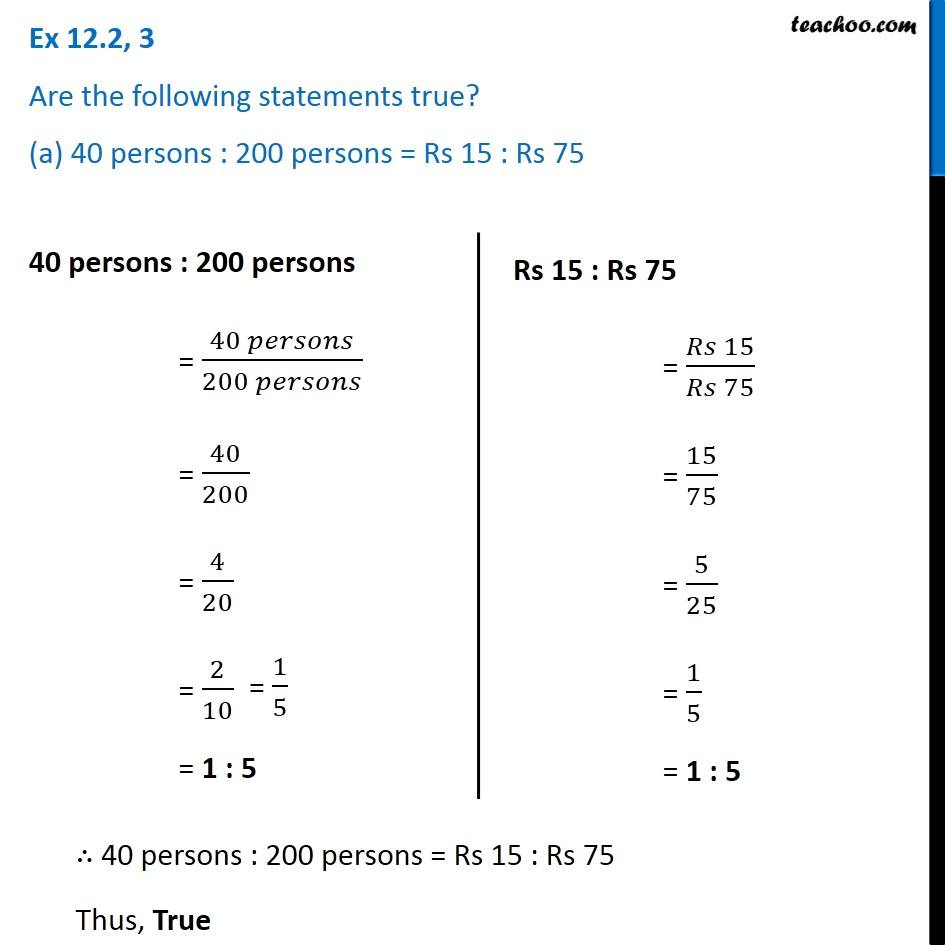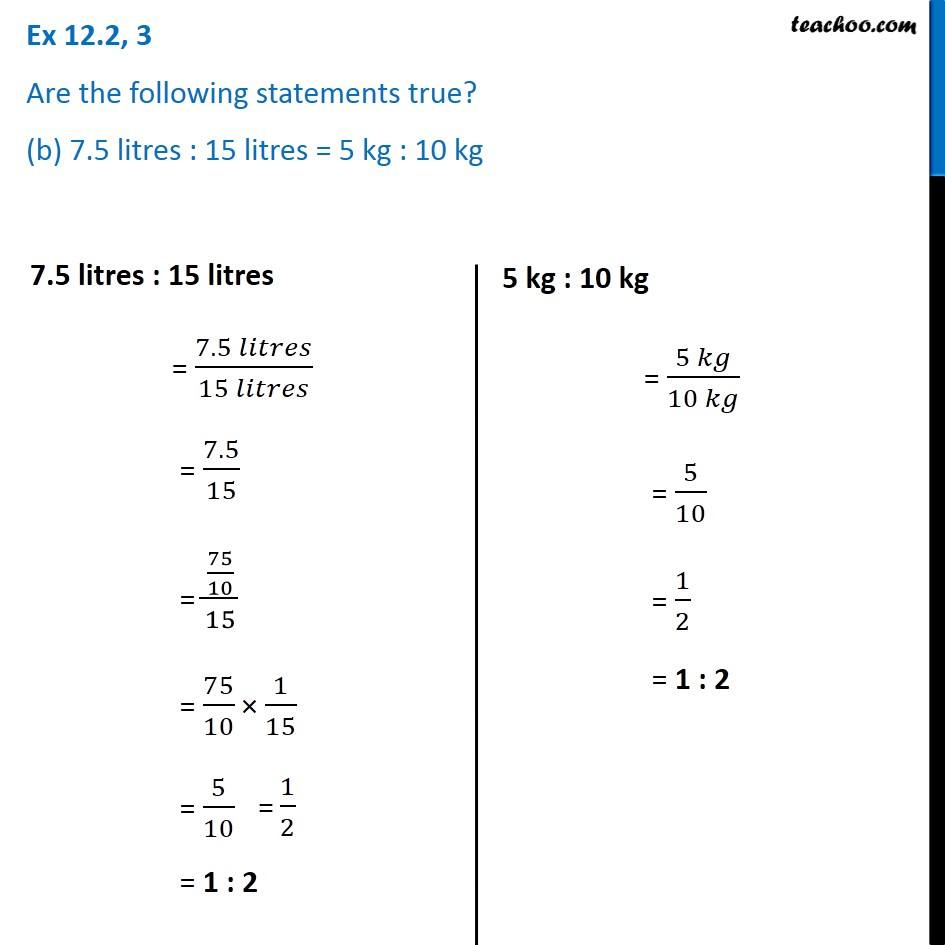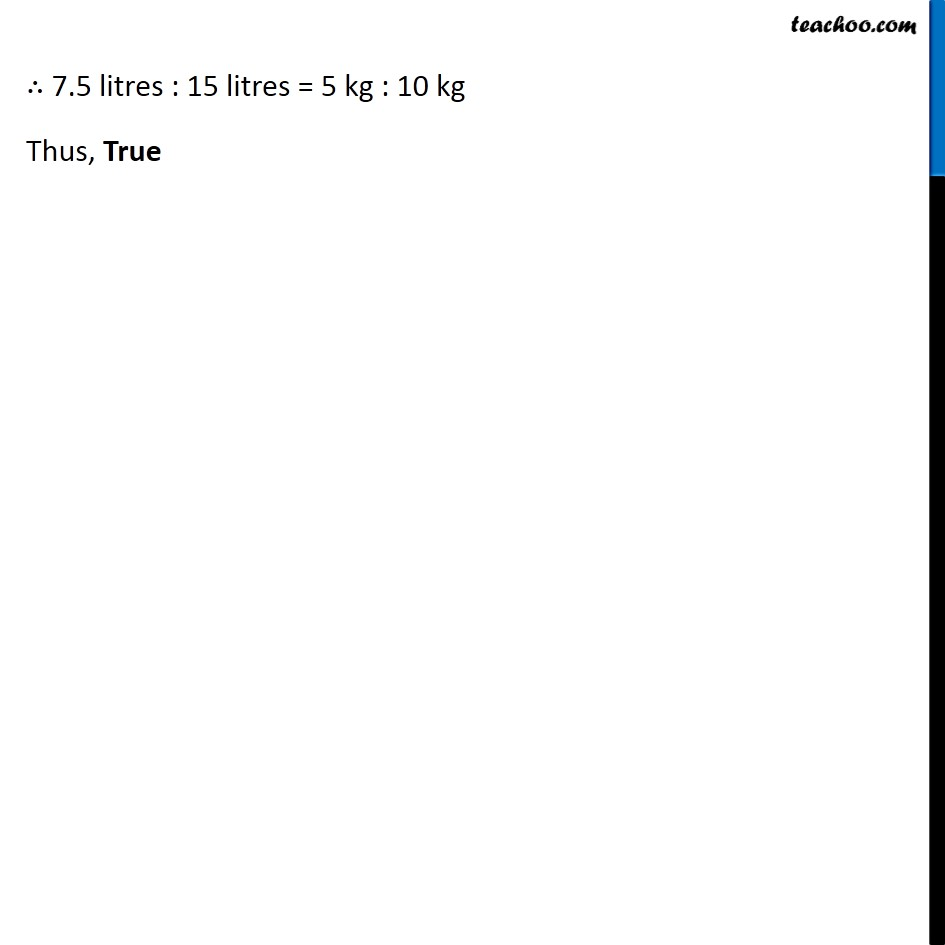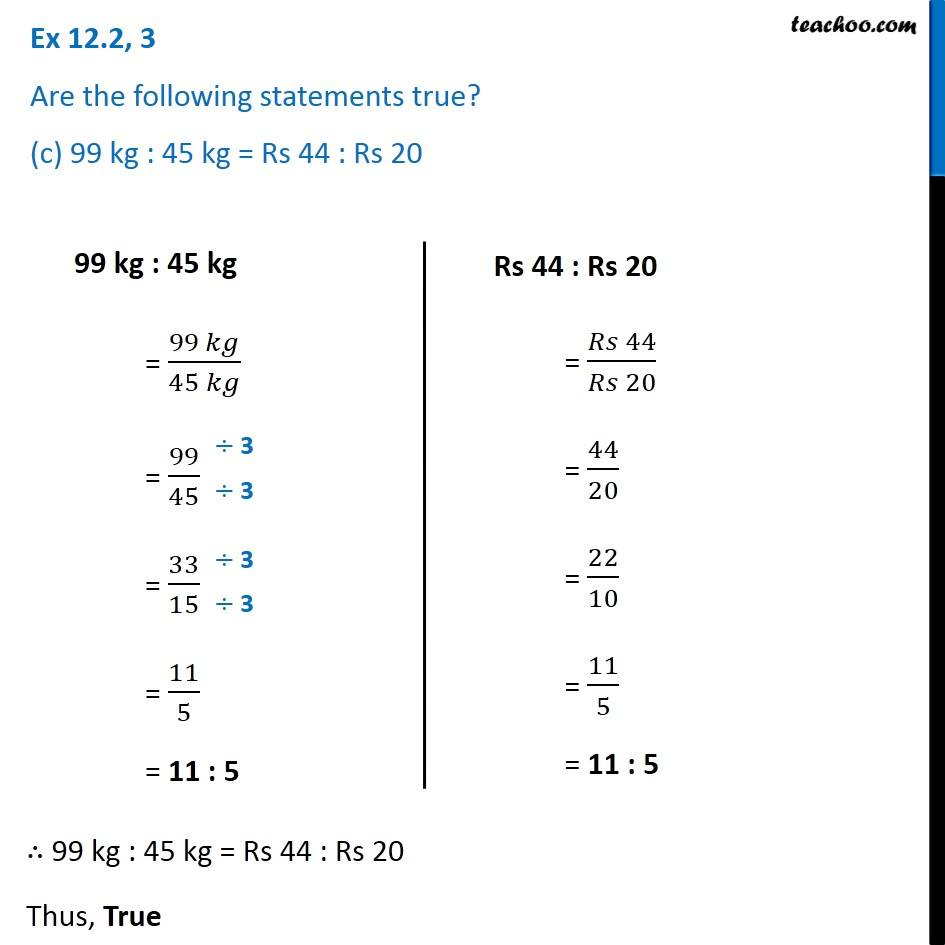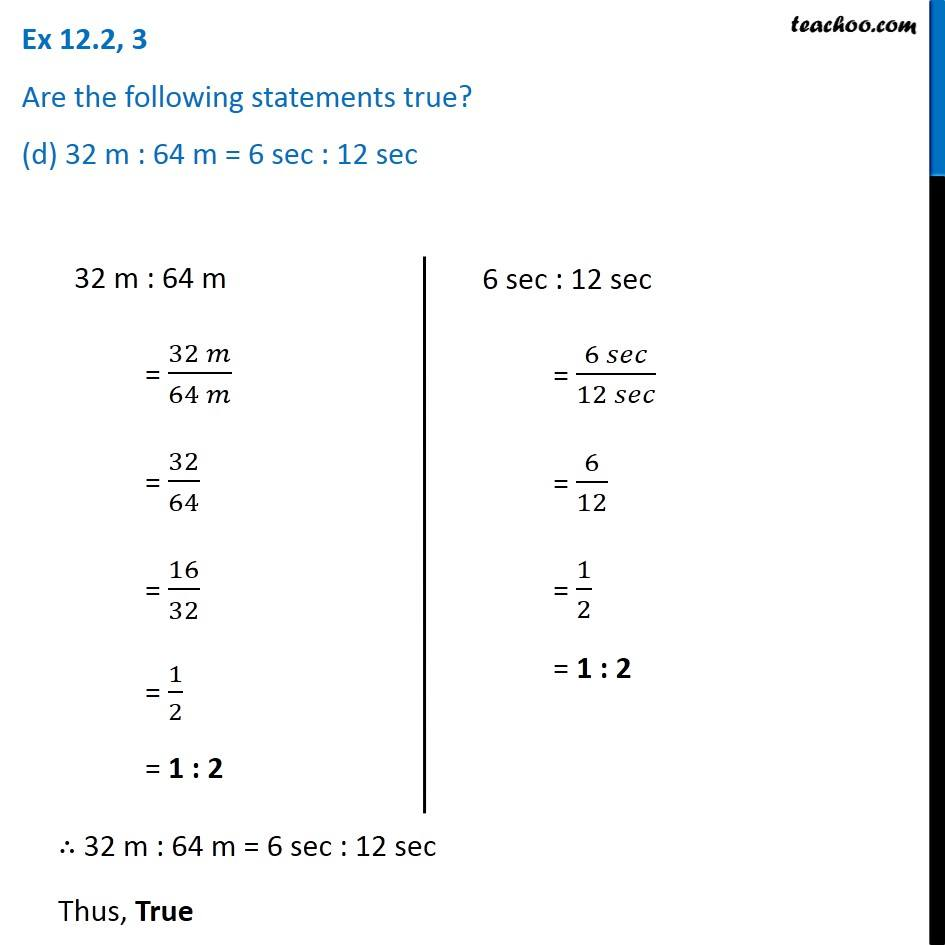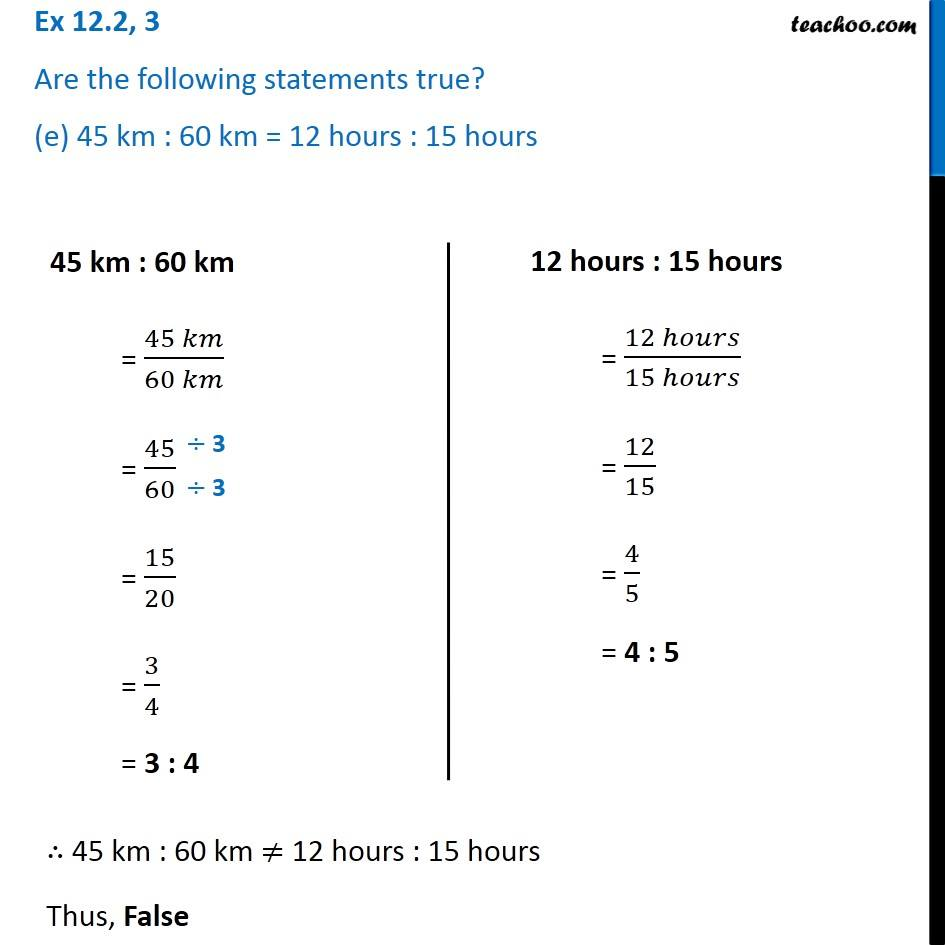Subscribe to our Youtube Channel - https://you.tube/teachoo

1. Chapter 12 Class 6 Ratio And Proportion
2. Serial order wise
3. Ex 12.2

Transcript

Ex 12.2, 3 Are the following statements true? (a) 40 persons : 200 persons = Rs 15 : Rs 75 Rs 15 : Rs 75 = (𝑅𝑠 15)/(𝑅𝑠 75) = 15/75 = 5/25 = 1/5 = 1 : 5 ∴ 40 persons : 200 persons = Rs 15 : Rs 75 Thus, True Ex 12.2, 3 Are the following statements true? (b) 7.5 litres : 15 litres = 5 kg : 10 kg7.5 litres : 15 litres = (7.5 𝑙𝑖𝑡𝑟𝑒𝑠)/(15 𝑙𝑖𝑡𝑟𝑒𝑠) = 7.5/15 = (75/10)/15 = 75/10 × 1/15 = 5/10 = 1 : 2 5 kg : 10 kg = (5 𝑘𝑔)/(10 𝑘𝑔) = 5/10 = 1/2 = 1 : 2 ∴ 7.5 litres : 15 litres = 5 kg : 10 kg Thus, True Ex 12.2, 3 Are the following statements true? (c) 99 kg : 45 kg = Rs 44 : Rs 2099 kg : 45 kg = (99 𝑘𝑔)/(45 𝑘𝑔) = 99/45 = 33/15 = 11/5 = 11 : 5 Rs 44 : Rs 20 = (𝑅𝑠 44)/(𝑅𝑠 20) = 44/20 = 22/10 = 11/5 = 11 : 5 ∴ 99 kg : 45 kg = Rs 44 : Rs 20 Thus, True Ex 12.2, 3 Are the following statements true? (d) 32 m : 64 m = 6 sec : 12 sec 6 sec : 12 sec = (6 𝑠𝑒𝑐)/(12 𝑠𝑒𝑐) = 6/12 = 1/2 = 1 : 2 ∴ 32 m : 64 m = 6 sec : 12 sec Thus, True Ex 12.2, 3 Are the following statements true? (e) 45 km : 60 km = 12 hours : 15 hours 45 km : 60 km = (45 𝑘𝑚)/(60 𝑘𝑚) = 45/60 = 15/20 = 3/4 = 3 : 4 12 hours : 15 hours = (12 ℎ𝑜𝑢𝑟𝑠)/(15 ℎ𝑜𝑢𝑟𝑠) = 12/15 = 4/5 = 4 : 5 ∴ 45 km : 60 km ≠ 12 hours : 15 hours Thus, False

Ex 12.2